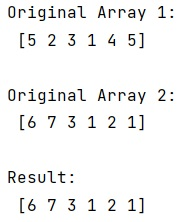# How to select elements of an array given condition?

Learn, how to select elements of an array given condition in Python?
Submitted by Pranit Sharma, on December 26, 2022

NumPy is an abbreviated form of Numerical Python. It is used for different types of scientific operations in python. Numpy is a vast library in python which is used for almost every kind of scientific or mathematical operation. It is itself an array which is a collection of various methods and functions for processing the arrays.

## Selecting elements of an array given condition

Suppose we are given two NumPy arrays and we need to select corresponding to the elements of another NumPy array that depends on some condition.

For this purpose, we will simply define an expression for writing the condition and filter out the data.

Suppose we need to filter out the data that is greater than 1 and less than 5, we will write an expression as follows:
( x>1) & (x < 5)

Let us understand with the help of an example,

## Python code to select elements of an array given condition

```# Import numpy
import numpy as np

# Creating two numpy arrays
arr1 = np.array([5, 2, 3, 1, 4, 5])
arr2 = np.array([6, 7, 3, 1, 2, 1])

# Display original arrays
print("Original Array 1:\n",arr1,"\n")
print("Original Array 2:\n",arr2,"\n")

# Defining a condition for filtering the data
res = arr2[(arr1)>1 & (arr1>5)]

# Display result
print("Result:\n",res)
```

Output: